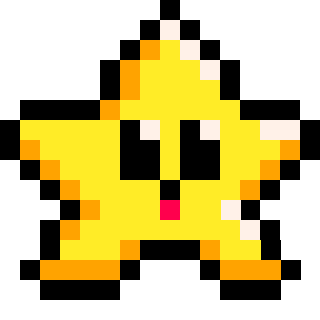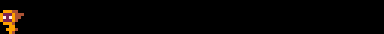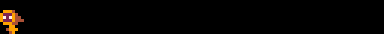# MID

``mid( a, b, c )``
 min "minimum" a a number b a number c a number

The mid(a, b, c) function is used to determine the number in the middle of three given numbers `a`, `b`, and `c`.For example, if we call `mid(10, 4, 20)`, the function will compare the three arguments and determine that `10` is the middle value. Therefore, it will return `10`.

``````middle = mid(10, 4, 20)
print(middle)  --prints 10``````

#### Using mid() to Create Limits

One way to use this function in your game, is to make sure a number stays within a certain range of numbers. For example, keeping a player within the screen's width. The player's x coordinate can be limited to the screen's left side of 0 and right side of 127.

If we call `mid()`, with the arguments of the player x, and the minimum and maximum of the allowed range, then the player's x will be limited to those minimum and maximum values. Here's an example:

``````left=0
right=127
x=60 --player

function _update()
--player movement
if btn(⬅️) then x-=1 end
if btn(➡️) then x+=1 end

x= mid(left,right,x)
end

function _draw()
spr(player_sprite,x,120)
end``````Notice how the player does move off the right side of the screen. This is to demonstrate that you need to remember that a sprite's X and Y coordinates are for the top-left corner of the sprite. So using mid above is still working to limit the player's X on the screen, because it is not letting the player's left side leave the range of the screen.

To fix this, remember to adjust the right side limit by the sprite's width like so:

``x= mid(left,right-8,x)``251

4 Oct 2023

`Font`